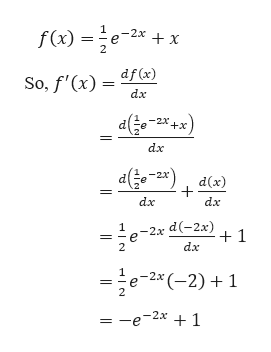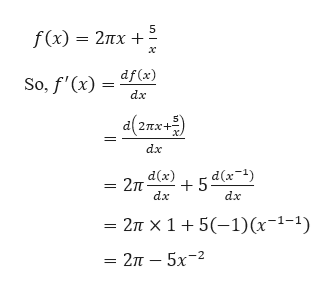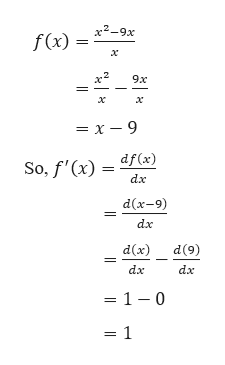unl(x)x6-2b. f(x) = 27x+x+ m_o% = ()S2. Find f'(x) for the following functions using the shortcuts we have learned thus far.

Question

please answer all parts of question 2help_outlineImage Transcriptioncloseunl (x) x6-2 b. f(x) = 27x+ x+ m_o% = ()S 2. Find f'(x) for the following functions using the shortcuts we have learned thus far. fullscreen
Step 1

Question No. 2

Part a

Given,help_outlineImage Transcriptionclosef(x)e-2x x So, f'(x) df(x) dax (x*x- a2xdx) dx dx da 1 dx 2 =e-2x (-2) 1 -e-2x 1 fullscreen
Step 2

Part b

Given,help_outlineImage Transcriptionclose5 f (x) — 2пх + х So, f'(x) df(x) dx al(знж ) 2пх+- dx d(x) 2п 5 dx (7-*)р dx — 2л х1+ 5(-1)(х-1-1) — 2п — 5х-2 fullscreen
Step 3

Part c

Given...help_outlineImage Transcriptionclosex2-9x f(x) х 9x х = x-9 So, f'(x)dfæ) dx d(x-9) dx d(x) d(9) dx da = 1 - 0 = 1 fullscreen

Want to see the full answer?

See Solution

Want to see this answer and more?

Our solutions are written by experts, many with advanced degrees, and available 24/7

See Solution
Tagged in

Derivative